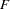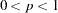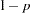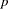The CANCORR Procedure
 PROC CANCORR Statement

PROC CANCORR <options> ;

The PROC CANCORR statement starts the CANCORR procedure and optionally identifies input and output data sets, specifies the analyses performed, and controls displayed output. Table 26.1 summarizes the options.

Table 26.1 Summary of PROC CANCORR Statement Options

Option

Description

Specify computational details

EDF=

specifies error degrees of freedom if input observations are regression residuals

MSTAT=

specifies the method of evaluating the multivariate test statistics

NOINT

omits intercept from canonical correlation and regression models

RDF=

specifies regression degrees of freedom if input observations are regression residuals

SINGULAR=

specifies the singularity criterion

Specify input and output data sets

DATA=

specifies input data set name

OUT=

specifies output data set name

OUTSTAT=

specifies output data set name containing various statistics

Specify labeling options

PARPREFIX=

specifies a prefix for naming residual variables

VNAME=

specifies a name to refer to VAR statement variables

VPREFIX=

specifies a prefix for naming VAR statement canonical variables

WNAME=

specifies a name to refer to WITH statement variables

WPREFIX=

specifies a prefix for naming WITH statement canonical variables

Control amount of output

ALL

produces simple statistics, input variable correlations, and canonical redundancy analysis

CORR

produces input variable correlations

NCAN=

specifies number of canonical variables for which full output is desired

NOPRINT

suppresses all displayed output

REDUNDANCY

produces canonical redundancy analysis

SHORT

suppresses default output from canonical analysis

SIMPLE

produces means and standard deviations

Request regression analyses

VDEP

requests multiple regression analyses with the VAR variables as dependents and the WITH variables as regressors

VREG

requests multiple regression analyses with the VAR variables as regressors and the WITH variables as dependents

WDEP

same as VREG

WREG

same as VDEP

Specify regression statistics

ALL

produces all regression statistics and includes these statistics in the OUTSTAT= data set

B

produces raw regression coefficients

CLB

produces 95% confidence interval limits for the regression coefficients

CORRB

produces correlations among regression coefficients

INT

requests statistics for the intercept when you specify the B, CLB, SEB, T, or PROBT option

PCORR

displays partial correlations between regressors and dependents

PROBT

displays probability levels forstatistics

SEB

displays standard errors of regression coefficients

SMC

displays squared multiple correlations andtests

SPCORR

displays semipartial correlations between regressors and dependents

SQPCORR

displays squared partial correlations between regressors and dependents

SQSPCORR

displays squared semipartial correlations between regressors and dependents

TB

displays standardized regression coefficients

T

displaysstatistics for regression coefficients

Following are explanations of the options that can be used in the PROC CANCORR statement (in alphabetic order).

ALL

displays simple statistics, correlations among the input variables, the confidence limits for the regression coefficients, and the canonical redundancy analysis. If you specify the VDEP or WDEP option, the ALL option displays all related regression statistics (unless the NOPRINT option is specified) and includes these statistics in the OUTSTAT= data set.

B

produces raw regression coefficients from the regression analyses.

CLB

produces the 95% confidence limits for the regression coefficients from the regression analyses.

CORR
C

produces correlations among the original variables. If you include a PARTIAL statement, the CORR option produces a correlation matrix for all variables in the analysis, the regression statistics (R square, RMSE), the standardized regression coefficients for both the VAR and WITH variables as predicted from the PARTIAL statement variables, and partial correlation matrices.

CORRB

produces correlations among the regression coefficient estimates.

DATA=SAS-data-set

names the SAS data set to be analyzed by PROC CANCORR. It can be an ordinary SAS data set or a TYPE=CORR, COV, FACTOR, SSCP, UCORR, or UCOV data set. By default, the procedure uses the most recently created SAS data set.

EDF=error-df

specifies the error degrees of freedom if the input observations are residuals from a regression analysis. The effective number of observations is the EDF= value plus one. If you have 100 observations, then specifying EDF=99 has the same effect as omitting the EDF= option.

INT

requests that statistics for the intercept be included when B, CLB, SEB, T, or PROBT is specified for the regression analyses.

MSTAT=FAPPROX | EXACT

specifies the method of evaluating the multivariate test statistics. The default is MSTAT=FAPPROX, which specifies that the multivariate tests are evaluated using the usual approximations based on thedistribution, as discussed in the section Multivariate Tests in Chapter 4, Introduction to Regression Procedures. Alternatively, you can specify MSTAT=EXACT to compute exact p-values for three of the four tests (Wilks’ lambda, the Hotelling-Lawley trace, and Roy’s greatest root) and an improvedapproximation for the fourth (Pillai’s trace). While MSTAT=EXACT provides better control of the significance probability for the tests, especially for Roy’s greatest root, computations for the exact p-values can be appreciably more demanding, and are in fact infeasible for large problems (many dependent variables). Thus, although MSTAT=EXACT is more accurate for most data, it is not the default method.

NCAN=number

specifies the number of canonical variables for which full output is desired. The number must be less than or equal to the number of canonical variables in the analysis.

The value of the NCAN= option specifies the number of canonical variables for which canonical coefficients and canonical redundancy statistics are displayed, and the number of variables shown in the canonical structure matrices. The NCAN= option does not affect the number of displayed canonical correlations.

If an OUTSTAT= data set is requested, the NCAN= option controls the number of canonical variables for which statistics are output. If an OUT= data set is requested, the NCAN= option controls the number of canonical variables for which scores are output.

NOINT

omits the intercept from the canonical correlation and regression models. Standard deviations, variances, covariances, and correlations are not corrected for the mean. If you use a TYPE=SSCP data set as input to the CANCORR procedure and list the variable Intercept in the VAR or WITH statement, the procedure runs as if you also specified the NOINT option. If you use NOINT and also create an OUTSTAT= data set, the data set is TYPE=UCORR.

NOPRINT

suppresses the display of all output. Note that this option temporarily disables the Output Delivery System (ODS). For more information, see Chapter 20, Using the Output Delivery System.

OUT=SAS-data-set

creates an output SAS data set to contain all the original data plus scores on the canonical variables. If you want to create a permanent SAS data set, you must specify a two-level name. The OUT= option cannot be used when the DATA= data set is TYPE=CORR, COV, FACTOR, SSCP, UCORR, or UCOV. For details about OUT= data sets, see the section Output Data Sets. See SAS Language Reference: Concepts for more information about permanent SAS data sets.

OUTSTAT=SAS-data-set

creates an output SAS data set containing various statistics, including the canonical correlations and coefficients and the multiple regression statistics you request. If you want to create a permanent SAS data set, you must specify a two-level name. For details about OUTSTAT= data sets, see the section Output Data Sets. See SAS Language Reference: Concepts for more information about permanent SAS data sets.

PCORR

produces partial correlations between regressors and dependent variables, removing from each dependent variable and regressor the effects of all other regressors.

PROBT

produces probability levels for thestatistics in the regression analyses.

RDF=regression-df

specifies the regression degrees of freedom if the input observations are residuals from a regression analysis. The effective number of observations is the actual number minus the RDF= value. The degrees of freedom for the intercept should not be included in the RDF= option.

REDUNDANCY
RED

produces canonical redundancy statistics.

PARPREFIX=name
PPREFIX=name

specifies a prefix for naming the residual variables in the OUT= data set and the OUTSTAT= data set. By default, the prefix is R_. The number of characters in the prefix plus the maximum length of the variable names should not exceed the current name length defined by the VALIDVARNAME= system option.

SEB

produces standard errors of the regression coefficients.

SHORT

suppresses all default output from the canonical analysis except the tables of canonical correlations and multivariate statistics.

SIMPLE
S

produces means and standard deviations.

SINGULAR=p
SING=p

specifies the singularity criterion, where. If a variable in the PARTIAL statement has an R square as large as(whereis the value of the SINGULAR= option) when predicted from the variables listed before it in the statement, the variable is assigned a standardized regression coefficient of 0, and the SAS log generates a linear dependency warning message. By default, SINGULAR=1E8.

SMC

produces squared multiple correlations andtests for the regression analyses.

SPCORR

produces semipartial correlations between regressors and dependent variables, removing from each regressor the effects of all other regressors.

SQPCORR

produces squared partial correlations between regressors and dependent variables, removing from each dependent variable and regressor the effects of all other regressors.

SQSPCORR

produces squared semipartial correlations between regressors and dependent variables, removing from each regressor the effects of all other regressors.

STB

produces standardized regression coefficients.

T

producesstatistics for the regression coefficients.

VDEP
WREG

requests multiple regression analyses with the VAR variables as dependent variables and the WITH Variables as regressors.

VNAME=label
VN=label

specifies a character constant to refer to variables from the VAR statement in the output. Enclose the constant in single or double quotes. If you omit the VNAME= option, these variables are referred to as the VAR variables. The number of characters in the label should not exceed the label length defined by the VALIDVARNAME= system option. For more information about the VALIDVARNAME= system option, see SAS Language Reference: Dictionary.

VPREFIX=name
VP=name

specifies a prefix for naming canonical variables from the VAR statement. By default, these canonical variables are given the names V1, V2, and so on. If you specify VPREFIX=ABC, the names are ABC1, ABC2, and so on. The number of characters in the prefix plus the number of digits required to designate the variables should not exceed the name length defined by the VALIDVARNAME= system option. For more information about the VALIDVARNAME= system option, see SAS Language Reference: Dictionary.

WDEP
VREG

requests multiple regression analyses with the WITH variables as dependent variables and the VAR variables as regressors.

WNAME=label
WN=label

specifies a character constant to refer to variables in the WITH statement in the output. Enclose the constant in single or double quotes. If you omit the WNAME= option, these variables are referred to as the WITH variables. The number of characters in the label should not exceed the label length defined by the VALIDVARNAME= system option. For more information about the VALIDVARNAME= system option, see SAS Language Reference: Dictionary.

WPREFIX=name
WP=name

specifies a prefix for naming canonical variables from the WITH statement. By default, these canonical variables are given the names W1, W2, and so on. If you specify WPREFIX=XYZ, the names are XYZ1, XYZ2, and so on. The number of characters in the prefix plus the number of digits required to designate the variables should not exceed the label length defined by the VALIDVARNAME= system option. For more information about the VALIDVARNAME= system option, see SAS Language Reference: Dictionary.Previous Page | Next Page | Top of Page